# Newton's method

Newton's method, also called the Newton-Raphson method, is a numerical root-finding algorithm: a method for finding where a function obtains the value zero, or in other words, solving the equation f(x) = 0. Most root-finding algorithms used in practice are variations of Newton's method. It is used widely to solve non-linear problems.

## Description of the algorithm

Suppose the function f(x) has a root at x = r. The idea behind Newton's method is that, if f(x) is a smooth function, its graph can be approximated around a point x = x0 by its tangent at x0. If the approximation is good enough, the point where the tangent crosses the x-axis must lie close to r. This suggests that if we choose the point x0 to lie somewhere close to r, the point where the tangent crosses the x-axis — we may call that point x1 — will lie even closer to r. The idea is illustrated in the following diagram:

Now suppose that x1 really does lie closer to r than x0. Then we can determine the tangent of f(x) at x = x1 to obtain a second point x2 that lies closer still. More generally, given xk, we can obtain a better approximation xk + 1.

Newton's method hinges on the fact that we can calculate the root xk + 1 of a tangent line directly from its straight-line equation y = ax + b, and that we can calculate a and b in terms of the function value f(xk) and the function's derivative at the same point,$f'(x_k)\,\!$. Put together, the relation between xk and xk + 1 can be expressed as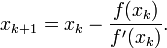$x_{k+1} = x_k - \frac{f(x_k)}{f'(x_k)}.$

This formula defines Newton's method. Using it to obtain an improved approximation is called to perform a Newton step, Newton update or Newton iteration. Newton's method consists of repeatedly using this formula to obtain a sequence of successively better estimates x1,x2,x3,... for r.

Let us illustrate Newton's method with a concrete numerical example. The golden ratio (φ ≈ 1.618) is the largest root of the polynomial f(x) = x2x − 1; to calculate this root, we can use the Newton iteration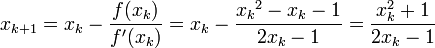$x_{k+1} = x_k - \frac{f(x_k)}{f'(x_k)} = x_k - \frac{{x_k}^2 - x_k - 1}{2x_k-1} = \frac{x_k^2 + 1}{2x_k - 1}$

with the initial estimate x0 = 1. Using double-precision floating-point arithmetic, which amounts to a precision of roughly 16 decimal digits, Newton's method produces the following sequence of approximations:

x0 = 1.0
x1 = 2.0
x2 = 1.6666666666666667
x3 = 1.6190476190476191
x4 = 1.6180344478216817
x5 = 1.618033988749989
x6 = 1.6180339887498947
x7 = 1.6180339887498949
x8 = 1.6180339887498949

Since the last iteration does not change the value, we can be reasonably sure to have obtained a value for the golden ratio that is correct to the precision used.

## Convergence analysis

In the description above, we relied on geometrical intuition to argue that Newton's method ought to produce a sequence of points xk that converge to the root r: that is, using the language of limits, we should be able to expect that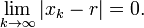$\lim_{k\to\infty} |x_k - r| = 0.$

We will show that this indeed is the case, under suitable conditions. In fact, we will be able to give a formula for how quickly the xk's approach r.

There is an important catch, however. In the geometrical description, we made the assumption that f(x) closely resembles a straight line at least locally (close to the root), and this assumption is in fact essential to the functioning of Newton's method. Most functions do not globally resemble lines: in general, function graphs contain curvature, stationary points, inflection points, and even discontinuities. Does Newton's method work in these cases?

The answer is maybe. If f(x) is ill-behaved or x0 lies very far from r, Newton's method may produce a sequence of numbers that jump back and forth erratically or even gradually move away from the root. If we are lucky, a value may eventually end up close to a root by chance. But if we are unlucky, Newton's method will fail to converge at all. For example, if Newton's method is used to solve the equation x3 − 5x = 0 with the initial guess x0 = 1, it will produce the endlessly repeating sequence 1, − 1,1, − 1,1,..., as shown in the figure below:

It may also happen that Newton's method converges to a root, but the "wrong" one. For example, if we attempt to calculate π as the smallest positive solution to sin(x) = 0, we must choose an initial value close to 3.14. Newton's method started near 0 would converge to 0; if started near 6.28, it would converge to , and so on. If we start with x0 close to π / 2, where the sine curve is horizontal, x1 may end up close to nπ for some enormous n.

But let us assume that the root r and the initial guess x0 both lie in a region where f(x) is well-behaved. In particular, we assume that f(x) is a repeatedly differentiable function in this region. Expanding f(x) in a Taylor series around x = xk gives$f(r) = 0 = f(x_k) + f'(x_k)(r-x_k) + \frac{1}{2} f''(\xi_k)(r-x_k)^2$

for some$\xi_k \in [r, x_k]$. Assuming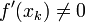$f'(x_k) \neq 0$, dividing through gives$0 = \frac{f(x_k)}{f'(x_k)} - x_k + r + \frac{1}{2} \frac{f''(\xi_k)}{f'(x_k)}(r-x_k)^2.$

Moving terms to the left hand side and substituting the Newton update expression for xk + 1 then gives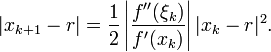$|x_{k+1}-r| = \frac{1}{2} \left| \frac{f''(\xi_k)}{f'(x_k)} \right| |x_k-r|^2.$

If the second derivative is bounded and the first derivative does not approach 0, the error at step k + 1 is hence roughly proportional to the square of the error at step k. Under these conditions, if for any k we have that | xkr | < 1 and | f''(ξn) / f'(ξn) | is small enough for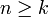$n \ge k$, subsequent iterations will reduce the error least quadratically (or at a second-order rate).

In other words, if the error at step k is 10ck, the error at the next step is roughly (10ck)2 = 10 − 2ck: each step roughly doubles the number of correct digits. This fast rate of convergence is characteristic for Newton's method. Looking back at the example of calculating the golden ratio, we can verify that the rate of convergence is quadratic in practice: the number of correct digits after the decimal point is 0, 0, 1, 2, 5, 12, and thereafter the convergence is limited by the finite precision which we used to carry out the arithmetic.

The second-order or quadratic convergence depends on | f''(ξk) / f'(ξk) | being small. There are two ways in which it may not be. The first possibility is that the second derivative in the numerator is large, which simply means that the function graph has a large amount of curvature and hence is poorly approximated by its tangent — we have already illustrated with examples how this may cause trouble.

The second possibility is that the first derivative in the denominator is very small; that is, if the tangent is nearly horizontal. In particular, the derivative becomes arbitrarily small if f'(r) = 0. This is an important case: it corresponds to f(x) having a multiple root at x = r. Newton's method can be proved to still converge if the root is a multiple root, but the rate of convergence in that case is merely linear: each iteration roughly increments, rather than multiplies, the number of correct digits by a fixed amount.

## Applications

So far we have only considered solving the equation f(x) = 0 in the real numbers, but Newton's method can be applied or generalized to various more complicated problems. To begin with, it can of course be used to solve the more general equation f(x) = g(x) by rewriting it as f(x) − g(x) = 0. It can also be used to calculate inverse functions. If f and f − 1 are inverse functions of each other, calculating x = f − 1(y) amounts to finding an x that solves the equation

f(x) − y = 0.

In many cases, Newton's method is a good choice for solving this equation. For example, the well known and very efficient Babylonian method for calculating square roots is equivalent to using Newton's method to solve the equation x2y = 0 for x (x2 being the inverse function of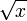$\sqrt x$).

Newton's method also works in the complex numbers; for example, it can be used to find complex roots of polynomials. Its behavior for complex analytic functions is similar to that for differentiable real functions; in particular, the rate of convergence is quadratic sufficiently close to a root. In the case of a polynomial with real coefficients, or any functions that only assumes real values for real input, the initial value must however be complex, or else the values generated by Newton's method never leave the real line. When Newton's method is applied to a polynomial with complex roots, the region of convergence around each root has a complicated boundary, which defines a fractal called a Newton fractal.

Newton's method can also be used as an optimization algorithm, to determine where a function obtains a local minimum or maximum. This amounts to solving the equation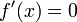$f'(x)=0\,\!$, which gives the Newton iteration$x_{k+1} = x_k - \frac{f'(x_k)}{f''(x_k)}.$

## Variations and hybrid methods

Newton's method may converge slowly with horizontal tangents. In addition, the speed of convergence depends upon the initial guess. These two factors make the method unsuitable as a black-box general-purpose root-finding algorithm. It is therefore typically combined with some mechanism for detecting and correcting convergence failure. One way to detect failure is count the number of iterations and break if the number exceeds some specified limit. Another technique is bracketing: the root is supplied along with known bounds for its location. Failure is signaled if the Newton iteration produces a value that lies outside the bounds.

In case of failure, it may be possible to recover by switching to a slower but safer algorithm, such as the bisection algorithm. After taking a few steps with the safe method, one can switch back to Newton's method and try again. This is called a hybrid method. Another option is the damped Newton's method, in which the derivative is multiplied by a damping factor α, with 0 < α < 1.

Newton's method requires that the derivative of the object function be known, but in some situations the derivative or Jacobian may be unavailable or prohibitively expensive to calculate. The cost can be higher still when Newton's method is used as an optimization algorithm, in which case the second derivative or Hessian is also needed.

An alternative in these situations is to use an approximation of the derivative or second-derivative, which leads to so-called quasi-Newton methods. The most common strategy is to use the function values from two successive iterations to calculate a finite difference approximation for the derivative. This is equivalent to the secant method and reduces the rate of convergence from 2 to 1.618 (more precisely, the golden ratio). An alternative is to compute a value for the derivative or second derivative accurately, but reusing the same value for several successive iterations.

Modifications of Newton's method can also lead to more specialized algorithms, such as the Durand-Kerner method which is used to find simultaneous roots of a polynomial.

## Computational complexity

Using Newton's method as described above, the time complexity of calculating a root of a function f(x) with n-digit precision, provided that a good initial approximation is known, is O((logn)F(n)) where F(n) is the cost of calculating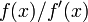$f(x)/f'(x)\,$ with n-digit precision.

If f(x) can be evaluated with variable precision, the algorithm can be improved by the self correcting method. Because of the "self-correcting" nature of Newton's method, meaning that it is unaffected by small perturbations once it has reached the stage of quadratic convergence, it is only necessary to use m-digit precision at a step where the approximation has m-digit accuracy. Hence, the first iteration can be performed with a precision twice as high as the accuracy of x0, the second iteration with a precision four times as high, and so on. If the precision levels are chosen suitably, only the final iteration requires$f(x)/f'(x)\,$ to be evaluated at full n-digit precision. Provided that F(n) grows superlinearly, which is the case in practice, the cost of finding a root is therefore only O(F(n)), with a constant factor close to unity.

This property of Newton's method makes it an essential tool for arbitrary-precision arithmetic. Division of large numbers is a good example. Multiplication of large numbers can be done efficiently using the fast Fourier transform, but there is no corresponding fast algorithm for division. However, division is the inverse operation of multiplication, and there is a Newton iteration for finding this inverse that uses only multiplication and subtraction. Therefore, by means of Newton's method, division can be performed nearly as fast as multiplication (in practice, about three times slower). Likewise, the time cost for computing square roots (as the inverse of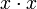$x \cdot x$) with Newton's method is proportional to that of multiplication.

## History

Newton's method was described by Isaac Newton in De analysi per aequationes numero terminorum infinitas (written in 1669, published in 1711 by William Jones) and in De metodis fluxionum et serierum infinitarum (written in 1671, translated and published as Method of Fluxions in 1736 by John Colson). However, his description differs substantially from the modern description given above: Newton applies the method only to polynomials. He does not compute the successive approximations xk, but computes a sequence of polynomials and only at the end, he arrives at an approximation for the root r. Finally, Newton views the method as purely algebraic and fails to notice the connection with calculus. Isaac Newton probably derived his method from a similar but less precise method by François Viète. The essence of Viète's method can be found in the work of Sharaf al-Din al-Tusi.

Newton's method was first published in 1685 in A Treatise of Algebra both Historical and Practical by John Wallis. In 1690, Joseph Raphson published a simplified description in Analysis aequationum universalis. Raphson again viewed Newton's method purely as an algebraic method and restricted its use to polynomials, but he describes the method in terms of the successive approximations xk instead of the more complicated sequence of polynomials used by Newton. Finally, in 1740, Thomas Simpson described Newton's method as an iterative methods for solving general nonlinear equations using fluxional calculus, essentially giving the description above. In the same publication, Simpson also gives the generalization to systems of two equations and notes that Newton's method can be used for solving optimization problems by setting the gradient to zero.